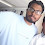# Pharma Engineering

For Engineer By Engineer

• ## Friday 9 December 2016

Hey visitors......!!!
The most basic thing that many engineers were aware of that is Boiling point varies with Vapour pressure, and i'll bet you that many of those Engineers donno the exact reason for this, and today i'll reveal that under hidden reason for that behavior of solvents.

For that you should be aware of some damn basic things like what does exactly Vapour pressure, Boiling point means.

What is Vapour Pressure ?

Vapour Pressure means the pressure exerted by the vapour on the surface of liquid at equilibrium, Usually vapour wont have any Vapour pressures, the vapour pressure is the property of solvents, every solvent will have their respective vapour pressures.

What is Boiling Point ?

Boiling point is nothing but a point of saturation where the vapour pressure of a solvent equals the atmospheric pressure, simply toluene is a solvent, whose boiling point is 110.6°C, and at that boiling point the vapour pressure of toluene will be 760 torr, [Dont get confused Torr means mmHg only]. And same is the case with every solvents boiling point.

How to Select a Condenser?
What Does a TR exactly means?
How to Calculate the Energy of Steam?

Right now, you got a clear idea of the Vapour Pressure & Boiling point, So now i'll start my show about describing the relation ship between Vapour Pressure and Boiling point, the only relation that describes the relation between these two parameters is Antonie Equation.

By using Antonie equation we can calculate the boiling point of solvent at different pressures, it also means that the boiling point of solvents will decrease with Vacuum, and we can calculate it theoretically. In fact, this is the first calculation that i've learned after joining the Pharma Industry.

The basic Antonie Equation is,

Log P = A - B / ( T + C ),

A, B, C are Antonie Constants,

P is Vapour Pressure, T is Boiling point.

Just remember one thing, while selecting the Antonie constants, there are many set of constants available, and they will vary with the units of Pressure that you  choose.

So, by now, if you are new to this calculation, this known equation will look something special to you,

Recommended Posts For You:

Many of the Pharma Operations will include "Distill off solvent under vacuum below temperature X°C" ,  here while they include word vacuum just because we can distill off the solvent below its regular boiling point.

I'll show you a small demo and Anyway i'll tell you how to use this practically,

"Distill off toluene under vacuum below 60°C with vacuum NLT 650 mmHg at the end of distillation",

This means the product that is present in our reaction mass is stable upto 60°C, and after that the product may degrade,

And here vacuum should be NLT 650 mmHg at the end of distillation, so now just try to calculate the boiling point at 650 mmHg,

For that you need to know the Antonie constants of Toluene,

Antonie constants data here

A =  6.95, B = 1344.8, C = 219.482,

the Vacuum should be NLT 650 mmHg, so the pressure should be P = 760 - 650 = 110 mmHg.

Log (110 ) = 6.95 - 1344.8 / ( T + 219.482 )

Solving for T, T = 54.4°C.

So, this means at 650 mmHg the boiling point of Toluene will be 54.4°C, which means it came down from 110.6°C to 54.4°C.

So, if you understand what i delivered above, just say cheers,

Still any doubts feel free to contact me,Hi! I am Ajay Kumar Kalva, Currently serving as the CEO of this site, a tech geek by passion, and a chemical process engineer by profession, i'm interested in writing articles regarding technology, hacking and pharma technology.

1.good.

2.1.how you calculated T value?

2.if you know the vapour pressure just insert in Antonie equation, and solve for T, that's it....!!!

Regards,
PHARMA ENGINEERING

3.thanks for ur valuable post.

4.Dear Ajay,
For selection of P value, do we have to substract required Vacuum (i.e.,650mmHg in case you have considered) from 760 mmHg or the atmospheric pressure at the given location (for example ~720mmHg in Hyderabad)??
Thanks

1.Dear Khan,

correct, you need to subtract from atnospheric pressure, i.e., 760 mmHg.

Regards,
AJAY K

2.Atmospheric pressure varies with sea level.Then, how do we consider that?

3.Dear Khan,

please go through the post, and try to calculate the atmospheric pressure at different locations based on height of sea level. Casually i've mentioned atmospheric pressure i.e., 760mmHg.

Regards,
AJAY K

5.Hello this is kedar,
How you find T value is 54.4,
By calculating the equation T value comes minus 874.96
Pls varifie the euation i;e log (110)=(6.95-1344.8)/(T+219.482)
Pls check.

6.Hello this is kedar,
How you calculate log110= (6.95-1344.8)/(T+219.482)
Its comes in minus value ,
But not 54.4

1.Hii Kedar,

You read the equation wrong, its Log(110) = 6.95 - (1344.8/(T+219.482)).

Now once try repeating the calculation, you will get it.

Best Regards,
AJAY K

7.Hello this is kedar,
Antonie constant are always same, if we take pressure unit is mmhg then its value is constant ? If we take pressure unit is kg/cm2 then whats it value ?pls reply.

1.Hii Kedar,

The constants will surely vary based on the units of pressure and temperature,
As we are much familiar with mmHg and C, i've given those.

Regards,
AJAY K

8.Hi
This Rachit
How we find out the values of A,B,C for different types of solvents

1.Hii Rachit,

Pl click on the link provided, 'ANTONIE CONSTANTS DATA HERE'.

Regards.

9.Hi,Hi! I am Ajay Kumar Kalva, owner of this site, a tech geek by passion, and a chemical process engineer by profession, i'm interested in writing articles regarding technology, hacking and pharma technology.